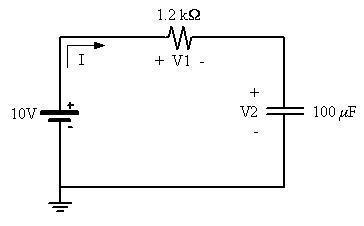# Calculating steady-state values in series RC circuit

• Engineering
• itlivesthere

## Homework Statement

Calculate the steady state values of the current, voltages V1 and V2 (defined by a period of time greater than five time constants). (Circuit in ASCII below).
+ V1 -
_________Res (1.2 kΩ)___________
| I => |
| | +
| + |
E 10V Cap (100 μF) V2
| - |
| | -
|------------------------------------------|
GND

## Homework Equations

τ=RC, E=IR, V= Vsource(1-e^-t/RC)

## The Attempt at a Solution

I'm a novice at electrical/electronics engineering, but I'm eager to learn all I can. Correct me if I'm wrong with the following:
I determined the time constant of this circuit is .12 seconds. As I understand correctly, it takes approximately five time constants for a capacitor to charge up to its steady-state voltage, which in this case equates to .6 seconds.
The current after it crosses the 1.2 kΩ resistor is calculated as 8.3 mA.
I'm very much stuck at this point... any advice?

Hi itlivesthere, welcome to PF.

You might want to put anything that depends upon horizontal spacing of characters (like ascii circuit diagrams) within [.code] [./code] delimiters (without the "." in each), and use a fixed width font to build the picture. You'll probably want to build the picture in a separate editor in which you can change the font accordingly, then cut & paste the result between the "code" delimiters.

Alternatively, upload a picture of your circuit, either drawn in a graphics program or snipped from a document. Here's an example showing what I think your circuit looks like:Now, on to your question. First you should recognize that this is a series circuit; all the components are connected end-to-end in a loop. In a series circuit the same current with the same value everywhere flows through each of the components. At steady state the current will be some constant value (and zero is a perfectly good value, too!). You stated your understanding that after some long time has passed (where engineers take "a long time" to mean more than 5 time constants!) that the capacitor will finish charging. What voltage will appear across the capacitor then?